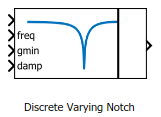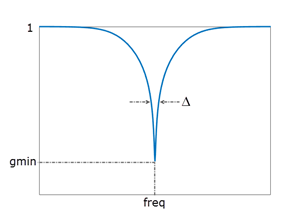# Discrete Varying Notch

Discrete-time notch filter with varying coefficients

•Libraries:
Control System Toolbox / Linear Parameter Varying

## Description

The block implements the Tustin discretization of a continuous-time notch filter with varying coefficients. Feed the continuous-time values of the notch frequency, minimum gain, and damping ratio to the freq, gmin, and damp input ports, respectively. These parameters control the notch depth and frequency of the continuous-time notch frequency as shown in the following illustration. The damping ratio damp controls the notch width Δ; larger damp means larger Δ.Use this block and the other blocks in the Linear Parameter Varying library to implement common control elements with variable parameters or coefficients. For more information, see Model Gain-Scheduled Control Systems in Simulink.

## Ports

### Input

expand all

Notch filter input signal

Continuous-time value of the notch frequency, specified in rad/s.

Continuous-time value of the gain at notch frequency, in absolute units. This value controls the notch depth. The notch filter has unit gain at low and high frequency. The gain is lowest at the notch frequency.

Continuous-time value of the damping ratio, specified as a positive scalar value. The damping ratio controls the notch width; the closer to 0, the steeper the notch.

### Output

expand all

Notch filter output signal.

## Parameters

expand all

Pre-warping frequency, specified as a positive scalar. Discretization of the continuous-time notch-filter transfer function can shift the notch frequency when it is close to the Nyquist frequency. To ensure that the continuous and discrete filters have matching frequency response near a particular frequency `w0`, set this parameter to `w0`. The default value `w0 = 0` corresponds to the bilinear (Tustin) transformation without pre-warp:

`$s=\frac{2}{{T}_{s}}\left(\frac{z-1}{z+1}\right),$`

where Ts is the block sample time, specified with the Sample time Ts parameter.

Block sample time, specified as a positive scalar. This block does not support inherited sample time, because it requires a specified sample time to compute the discretization of the notch filter.

## Version History

Introduced in R2017b